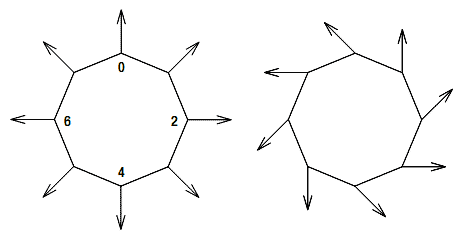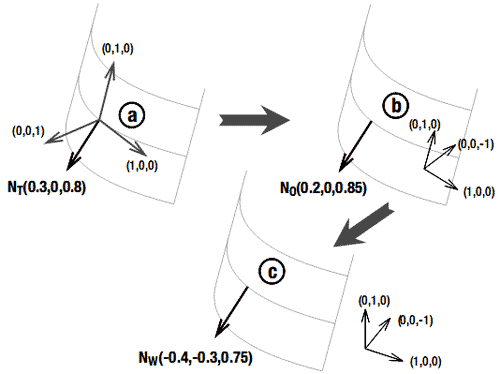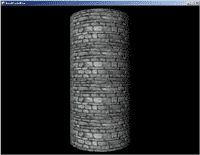# 5.14 通过切线空间的凹凸映射添加逐像素细节

## 问题## 解决方案## 工作原理

### 定义自定义顶点格式

public struct VertPosTexNormTan
{
public Vector3 Position;
public Vector2 TexCoords;
public Vector3 Normal;
public Vector3 Tangent;
public VertPosTexNormTan(Vector3 Position, Vector2 TexCoords, Vector3 Normal, Vector3 Tangent)
{
this.Position = Position;
this.TexCoords = TexCoords;
this.Normal = Normal;
this.Tangent = Tangent;
}

public static readonly VertexElement[] VertexElements =
{
new VertexElement(0, 0, VertexElementFormat.Vector3, VertexElementMethod.Default, VertexElementUsage.Position, 0),
new VertexElement(0, sizeof(float)*3, VertexElementFormat.Vector2, VertexElementMethod.Default, VertexElementUsage.TextureCoordinate, 0),
new VertexElement(0, sizeof(float)*(3+2), VertexElementFormat.Vector3, VertexElementMethod.Default, VertexElementUsage.Normal, 0),
new VertexElement(0, sizeof(float)*(3+2+3), VertexElementFormat.Vector3, VertexElementMethod.Default, VertexElementUsage.Tangent, 0),
};

public static readonly int SizeInBytes = sizeof(float) * (3 + 2 + 3 + 3);
}

### 定义每个顶点的法线和切线

private void InitVertices()
{
List<VertPosTexNormTan> verticesList = new List<VertPosTexNormTan>();

int detail = 20;
float height = 8;
for (int i = 0; i < detail + 1; i++)
{
float angle = MathHelper.Pi * 2.0f / (float)detail * (float)i;
Vector3 baseVector = Vector3.Transform(Vector3.Forward, Matrix.CreateRotationY(angle));
Vector3 posLow = baseVector * radius;
posLow.Y = -height / 2.0f;
Vector3 posHigh = posLow;
posHigh.Y += height;
Vector2 texCoordLow = new Vector2(angle / (MathHelper.Pi * 2.0f), 1);
Vector2 texCoordHigh = new Vector2(angle / (MathHelper.Pi * 2.0f), 0);

verticesList.Add(new VertPosTexNormTan(posLow, texCoordLow, Vector3.Zero, new Vector3(0, 1, 0)));
verticesList.Add(new VertPosTexNormTan(posHigh, texCoordHigh, Vector3.Zero, new Vector3(0, 1, 0)));
}
vertices = verticesList.ToArray();
}

vertices = InitVertices();
indices = InitIndices(vertices);
vertices = GenerateNormalsForTriangleList(vertices, indices); 

### XNA-to-HLSL变量

float4x4 xWorld;
float4x4 xView;
float4x4 xProjection;
float3 xLightDirection;
float xTexStretch;
Texture xTexture;
sampler TextureSampler = sampler_state
{
texture = <xTexture> ;
magfilter = LINEAR;
minfilter = LINEAR;
mipfilter=LINEAR;
};

Texture xBumpMap;
sampler BumpMapSampler = sampler_state
{
texture = <xBumpMap> ;
magfilter = LINEAR;
minfilter = LINEAR;
mipfilter=LINEAR;
};

struct BMVertexToPixel
{
float4 Position : POSITION;
float2 TexCoord : TEXCOORD0;
float3x3 TTW : TEXCOORD1;
};

struct BMPixelToFrame
{
float4 Color : COLOR0;
} 

BMVertexToPixel BMVertexShader(float4 inPos: POSITION0, float3 inNormal: NORMAL0, float2 inTexCoord: TEXCOORD0, float3 inTangent: TANGENT0)
{
BMVertexToPixel Output = (BMVertexToPixel)0;
float4x4 preViewProjection = mul(xView, xProjection);
float4x4 preWorldViewProjection = mul(xWorld, preViewProjection);

Output.Position = mul(inPos, preWorldViewProjection);
Output.TexCoord = inTexCoord; return Output;
} 

float3 Binormal = normalize(cross(inTangent,inNormal));

float3x3 tangentToObject;
tangentToObject = normalize(Binormal);
tangentToObject = normalize(inTangent);
tangentToObject = normalize(inNormal);

float3x3 tangentToWorld = mul(tangentToObject, xWorld);
Output.TTW = tangentToWorld; 

BMPixelToFrame BMPixelShader(BMVertexToPixel PSIn) : COLOR0
{
BMPixelToFrame Output = (BMPixelToFrame)0;
float3 bumpColor = tex2D(BumpMapSampler, PSIn.TexCoord*xTexStretch);
float3 normalT = (bumpColor - 0.5f)*2.0f;
float3 normalW = mul(normalT, PSIn.TTW);
float lightFactor = dot(-normalize(normalW),normalize(xLightDirection));
float4 texColor = tex2D(TextureSampler, PSIn.TexCoord*xTexStretch);
Output.Color = lightFactor*texColor;

return Output;
} 

normalT值为(0,0,1)表示偏离法线等于默认法线(即切线空间中的z轴方向)。normalT值为(0,0.7,0.7)表示法线在默认法线和切线（切线空间的y轴方向）间的45度角方向。

## 代码

### HLSL部分

BMVertexToPixel BMVertexShader(float4 inPos: POSITION0, float3 inNormal: NORMAL0, float2 inTexCoord: TEXCOORD0, float3 inTangent: TANGENT0)
{
BMVertexToPixel Output = (BMVertexToPixel)0;
float4x4 preViewProjection = mul(xView, xProjection);
float4x4 preWorldViewProjection = mul(xWorld, preViewProjection);
Output.Position = mul(inPos, preWorldViewProjection);
Output.TexCoord = inTexCoord;
float3 Binormal = cross(inTangent,inNormal);
float3x3 tangentToObject;
tangentToObject = normalize(Binormal);
tangentToObject = normalize(inTangent);
tangentToObject = normalize(inNormal);
float3x3 tangentToWorld = mul(tangentToObject, xWorld);
Output.TTW = tangentToWorld;

return Output;
} 

BMPixelToFrame BMPixelShader(BMVertexToPixel PSIn) : COLOR0
{
BMPixelToFrame Output = (BMPixelToFrame)0;
float3 bumpColor = tex2D(BumpMapSampler, PSIn.TexCoord*xTexStretch);
float3 normalT = (bumpColor - 0.5f)*2.0f;
float3 normalW = mul(normalT, PSIn.TTW);
float lightFactor = dot(-normalize(normalW),normalize(xLightDirection));
float4 texColor = tex2D(TextureSampler, PSIn.TexCoord*xTexStretch);
Output.Color = lightFactor*texColor;

return Output;
} 

### XNA部分

XNA项目要提供每个顶点的切线，切线向量垂直于法线，生成这些顶点的方法前面已经写过了。

effect.CurrentTechnique = effect.Techniques["BumpMapping"];
effect.Parameters["xWorld"].SetValue(Matrix.Identity);
effect.Parameters["xView"].SetValue(fpsCam.ViewMatrix);
effect.Parameters["xProjection"].SetValue(fpsCam.ProjectionMatrix);
effect.Parameters["xTexture"].SetValue(myTexture);
effect.Parameters["xBumpMap"].SetValue(myBumpMap);
effect.Parameters["xLightDirection"].SetValue(lightDirection);
effect.Parameters["xTexStretch"].SetValue(4.0f);

effect.Begin();
foreach (EffectPass pass in effect.CurrentTechnique.Passes)
{
pass.Begin();
device.VertexDeclaration = myVertexDeclaration;
device.DrawUserIndexedPrimitives<VertPosTexNormTan>(PrimitiveType.TriangleList, vertices, 0, vertices.Length, indices, 0, indices.Length/3);
pass.End();
}
effect.End();

## 相反的方法

struct BMVertexToPixel
{
float4 Position : POSITION;
float2 TexCoord : TEXCOORD0;
float3 LightDirT: TEXCOORD1;
}; 

float3 vectorW = mul(vectorT, tangentToWorld);

float3 vectorT = mul(tangentToWorld, vectorW);

Output.LightDirT = mul(tangentToWorld, xLightDirection);

float3 bumpColor = tex2D(BumpMapSampler, PSIn.TexCoord*xTexStretch);
float3 normalT = (bumpColor - 0.5f)*2.0f;
float lightFactor = dot(-normalize(normalT), normalize(PSIn.LightDirT));2006 - 2023，推荐分辨率1024*768以上，推荐浏览器Chrome、Edge等现代浏览器，截止2021年12月5日的访问次数：1872万9823 站长邮箱